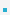2. Pointers to StructuresStructures are copied element wise.For large structures, it is more efficient to pass pointers.
 void foo(struct point ∗pp); struct point pt; foo(&pt)Members can be accesses from structure pointers using ‘->’ operator.
 struct point p = {10,20}; struct point ∗pp = &p ; pp−>x = 10; /∗ changes p.x ∗/ int y = pp−>y;         /∗ same as y=p.y ∗/Other ways to access structure members? Why is the () required?
 struct point p = {10,20}; struct point ∗pp = &p ; (∗pp).x = 10;              /∗ changes p.x ∗/ int y = (∗pp).y; /∗ same as y=p.y ∗/

 이전페이지 / 3 / 다음페이지